# hashmap扩容时死循环问题

public V put(K key, V value)
{
......
//计算Hash值
int hash = hash(key.hashCode());
int i = indexFor(hash, table.length);
//各种校验吧
for (Entry<K,V> e = table[i]; e != null; e = e.next) {
Object k;
if (e.hash == hash && ((k = e.key) == key || key.equals(k))) {
V oldValue = e.value;
e.value = value;
e.recordAccess(this);
return oldValue;
}
}
modCount++;
//该key不存在，需要增加一个结点
return null;
}

void addEntry(int hash, K key, V value, int bucketIndex)
{
Entry<K,V> e = table[bucketIndex];
table[bucketIndex] = new Entry<K,V>(hash, key, value, e);
//查看当前的size是否超过了我们设定的阈值threshold，如果超过，需要resize
if (size++ >= threshold)
resize(2 * table.length);//扩容都是2倍2倍的来的，
}

void resize(int newCapacity)
{
Entry[] oldTable = table;
int oldCapacity = oldTable.length;
......
//创建一个新的Hash Table
Entry[] newTable = new Entry[newCapacity];
//将Old Hash Table上的数据迁移到New Hash Table上
transfer(newTable);
table = newTable;
}

void transfer(Entry[] newTable)
{
Entry[] src = table;
int newCapacity = newTable.length;
//下面这段代码的意思是：
//  从OldTable里摘一个元素出来，然后放到NewTable中
for (int j = 0; j < src.length; j++) {
Entry<K,V> e = src[j];
if (e != null) {
src[j] = null;
do {
Entry<K,V> next = e.next;
int i = indexFor(e.hash, newCapacity);
e.next = newTable[i];
newTable[i] = e;
e = next;
} while (e != null);
}
}
}

do循环里面的是最能说明问题的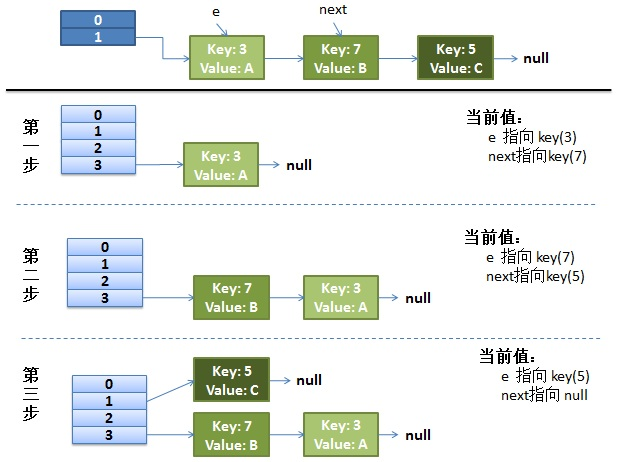do {
Entry<K,V> next = e.next; // <--假设线程一执行到这里就被调度挂起了
int i = indexFor(e.hash, newCapacity);
e.next = newTable[i];
newTable[i] = e;
e = next;
} while (e != null);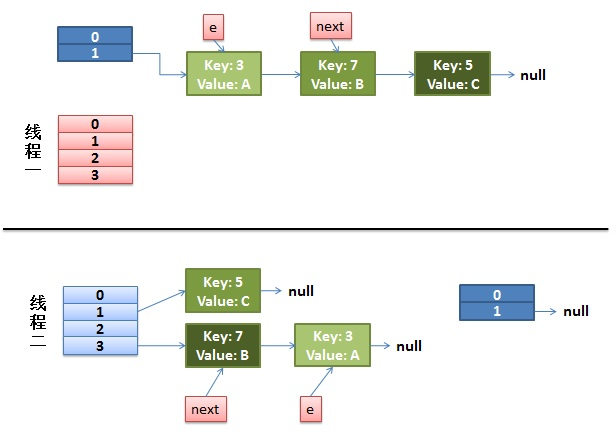2）线程一被调度回来执行。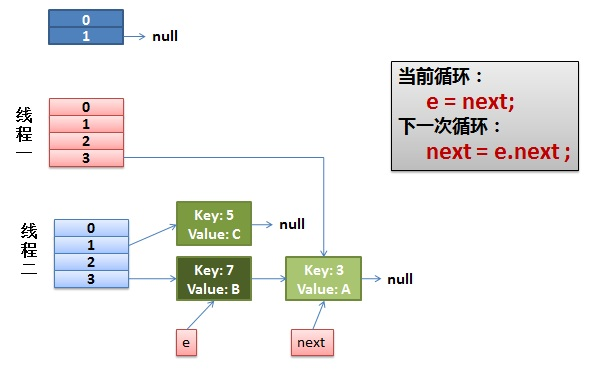3）一切安好。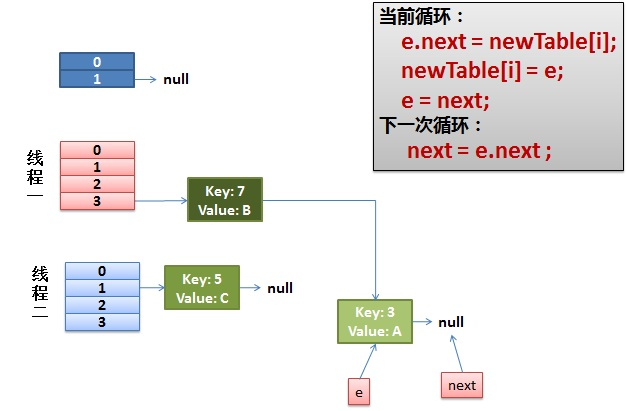4）环形链接出现。

e.next = newTable[i] 导致 key(3).next 指向了 key(7)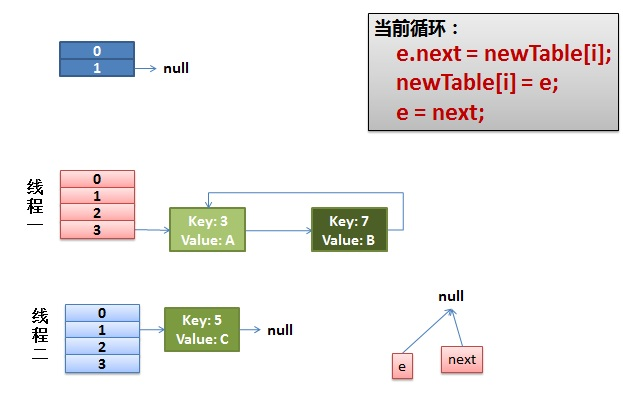12-077760
05-26128407-061992
06-16386
05-11506
08-217145
11-22534
07-184770
07-143980
03-1569